# Concept Summary: Time Series Interval Extraction Pt 1¶

When working with time series data, we are sometimes interested in data that lie within specified boundary values, and finding the corresponding time segments for this data.

We’ll tackle this topic in three parts:

• The motivation for interval extraction with time series data.

• The mechanics behind the Interval Extraction recipe in the time series preparation plugin.

• Demo: how to use the recipe in Dataiku DSS.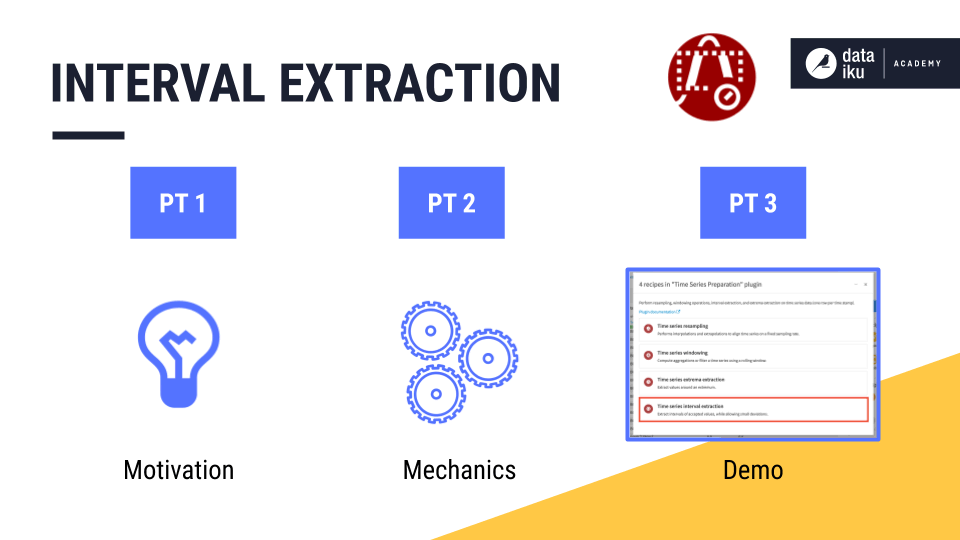## The Motivation for Interval Extraction¶

Imagine a factory where the production line follows a normal distribution. Every minute, the line produces, on average, “mu” items with some standard deviation “sigma”.

Suppose we want to flag time intervals during which the factory produced more than three standard deviations above or below the average. We could then investigate the conditions associated with these anomalies. How could we identify these time intervals with our existing tools in Dataiku DSS?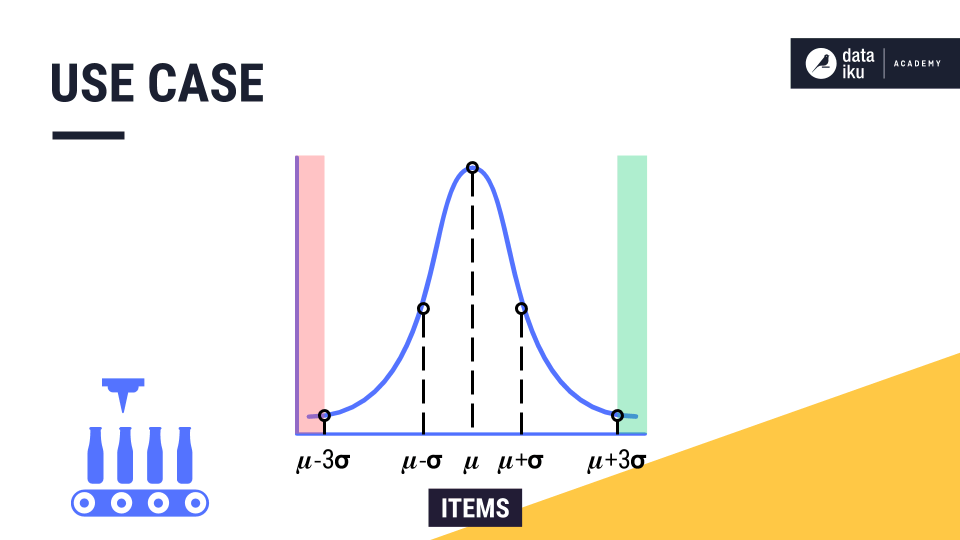To build up to this use case, let’s return to our familiar example of t-shirt revenue, plotted on the y-axis, across time, plotted on the x-axis. Imagine we want to identify intervals of typical days, that is, days when the revenue lies between $25,000 and$35,000.

For each row of the data, we ask one simple question. Does the value lie within our threshold range?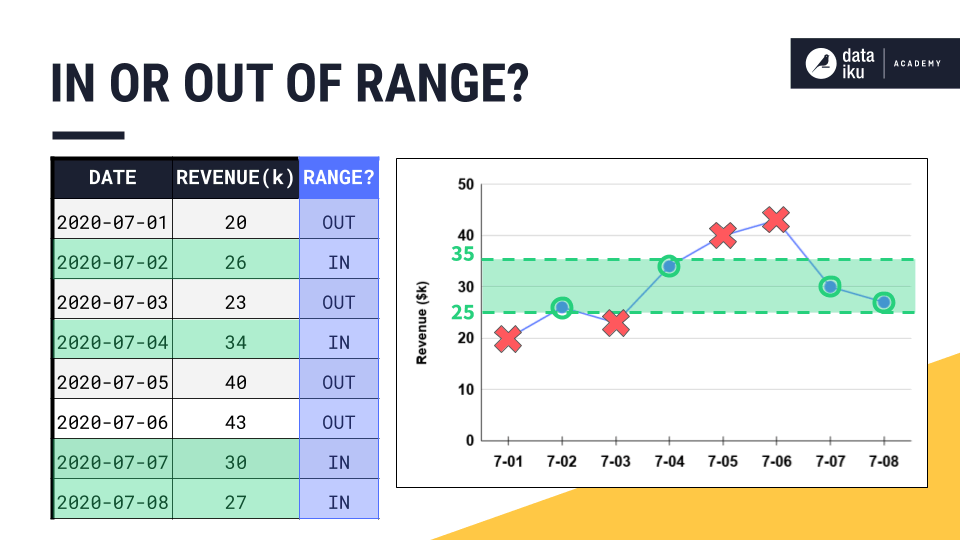To achieve this, you could use a Filter recipe or a Filter processor in a Prepare recipe, for example. But with time series, we sometimes want to attach conditions in addition to setting an upper and lower bound.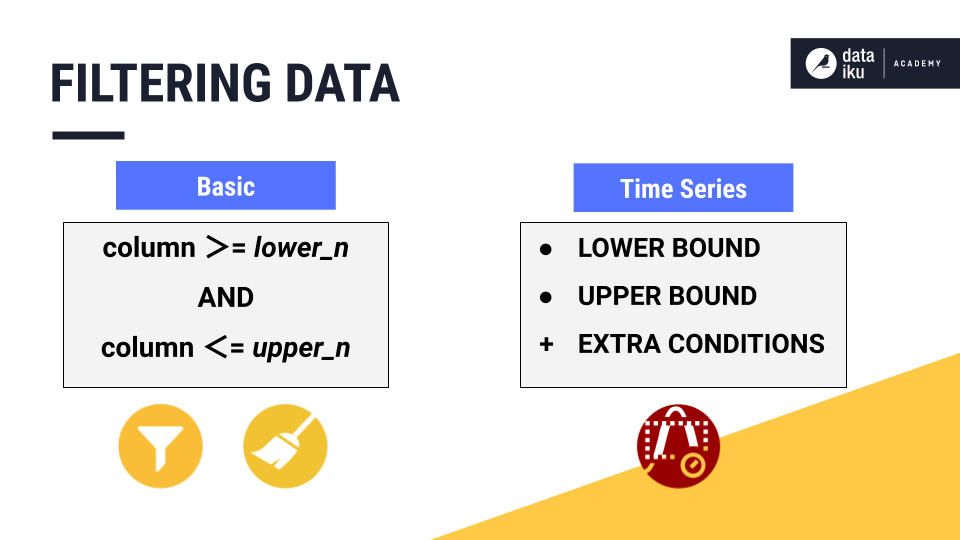In this lesson, we’ll discuss three advantages that the Interval Extraction recipe in the Time Series preparation plugin offers above the basic kind of filtering.

For example, we may want to consider:

• keeping track of retained intervals

• defining an acceptable deviation period, and

• defining a minimum segment deviation.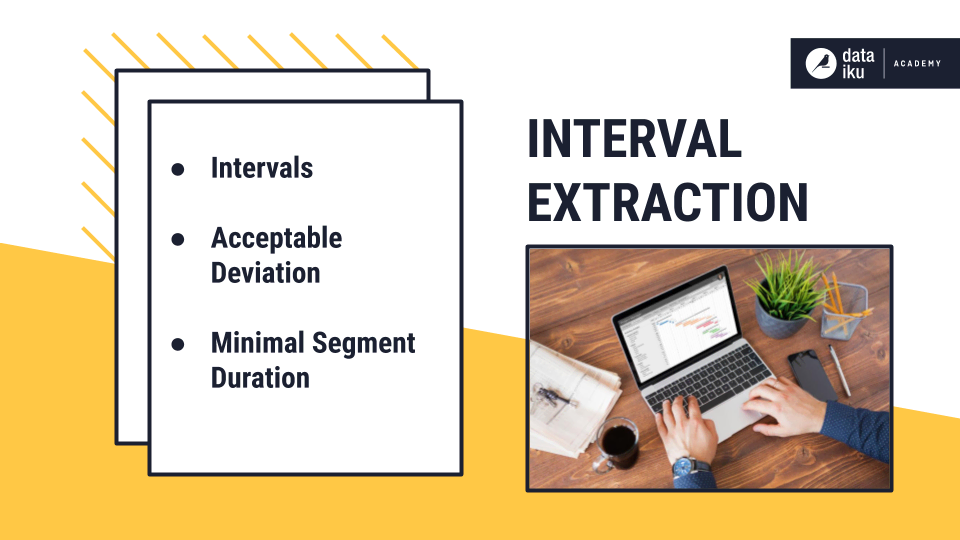### Intervals¶

Remember that time series are not made of independent observations. Rather, these observations are dependent on time. This means that the valid intervals – the time periods containing observations that lie between the upper and lower bound – represent valuable information.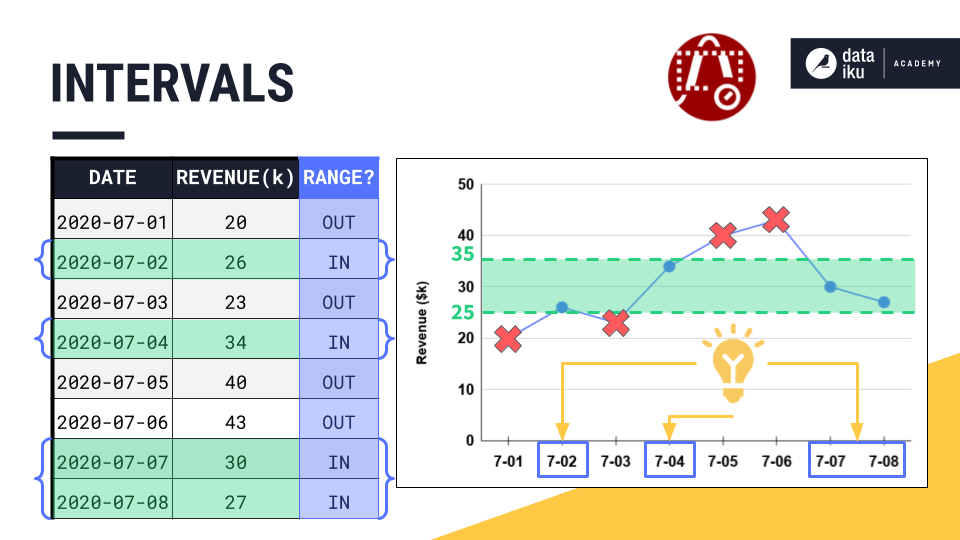For this reason, we often want to keep track of those valid intervals, by using a different ID, for each interval, as we move along the time series from start to finish. Once we have the intervals recorded in our dataset, we can define new features to use for modeling or for further analysis.

For example, we can use a Window recipe to calculate the average length of an interval or the elapsed time since the previous interval.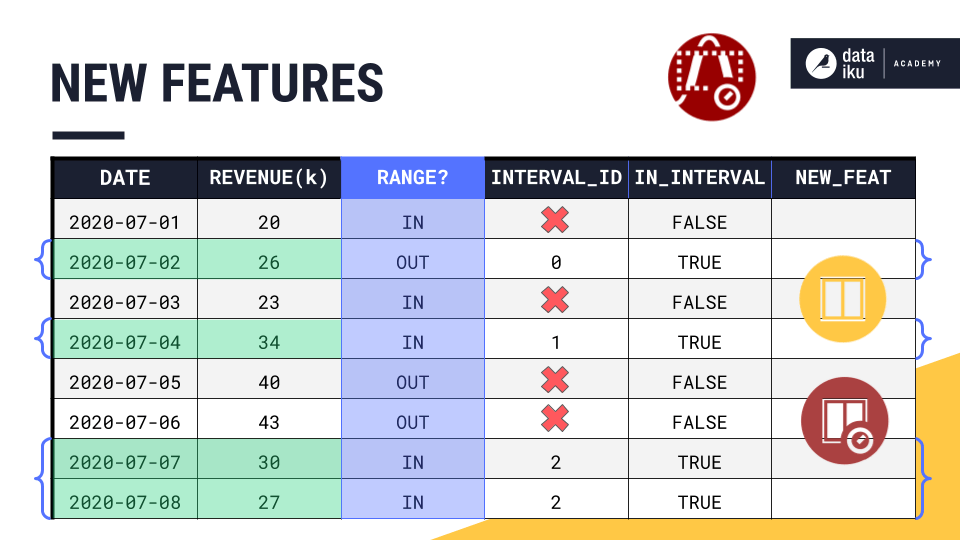### Acceptable Deviation¶

Setting an acceptable deviation can be particularly useful in cases where volatility exists in our time series, and we want our time intervals to retain brief deviations from the threshold range, while excluding longer deviations.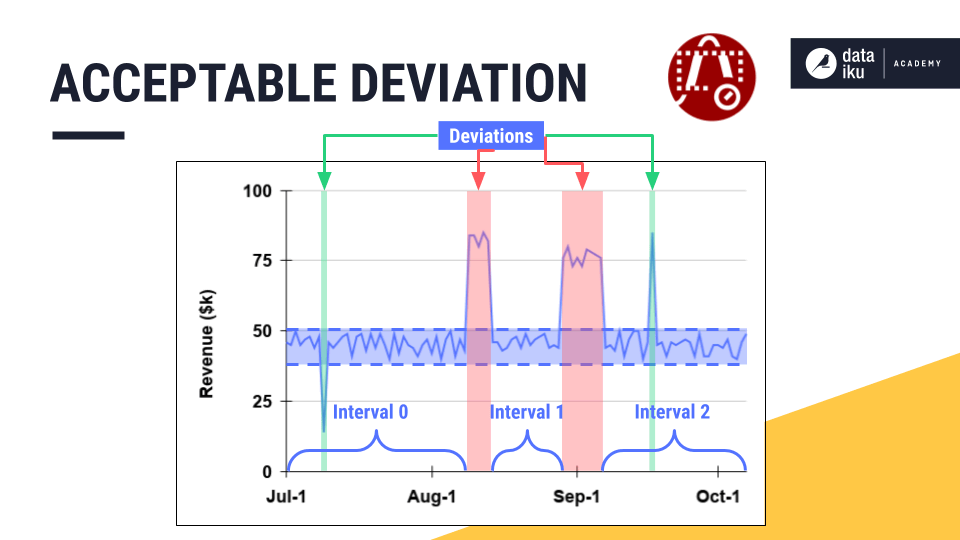In our example, consider that the point for July 3rd is out of the threshold range. However, the previous timestamp is in the threshold range. And the very next timestamp is also back in the threshold range. So the time series skipped out of range for just one day.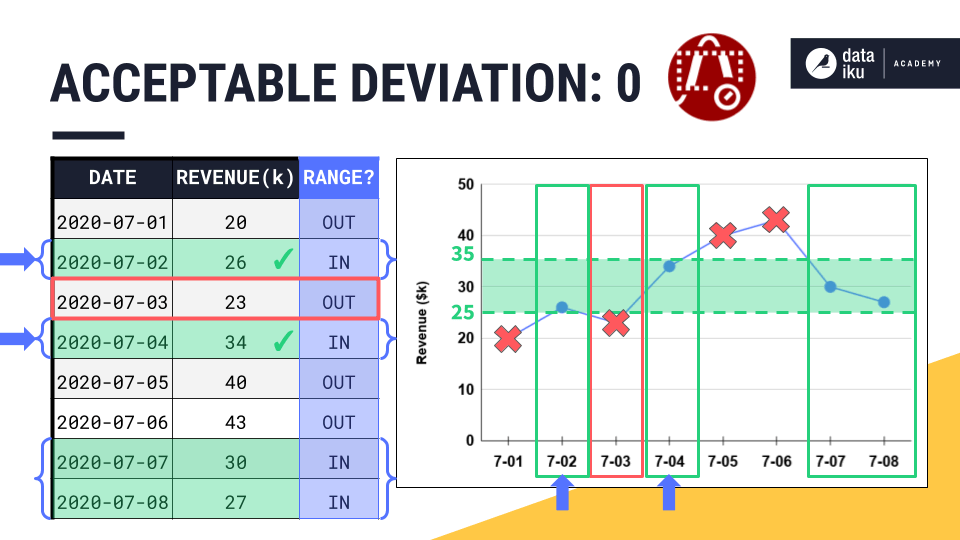By setting an acceptable deviation of 1 day, we would absorb the point for July 3rd, as well as the point for July 1st, into one single time interval with its neighbors.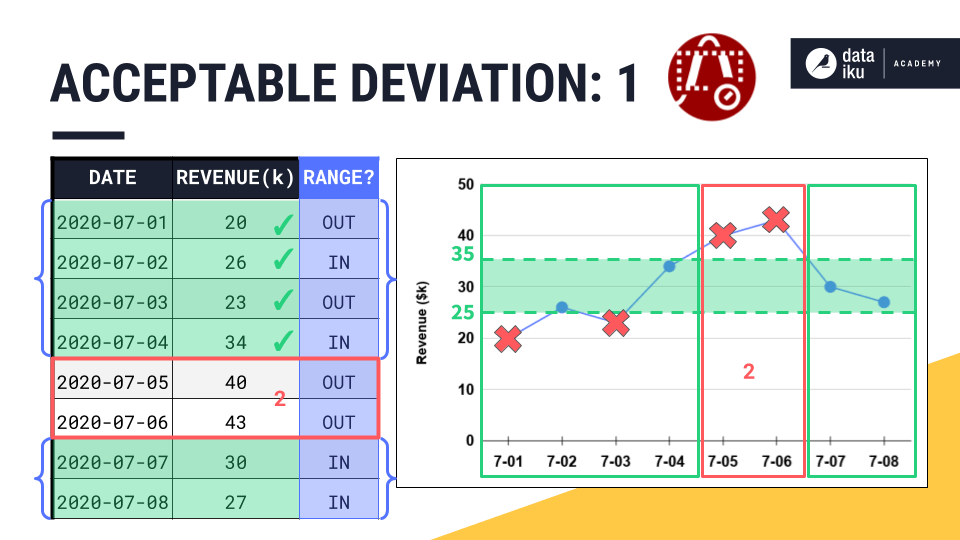However, the points for July 5th and 6th are outside the threshold range for a time period that is longer than the acceptable deviation. We would need an acceptable deviation of at least 2 days if we wanted to include these points in an interval ID.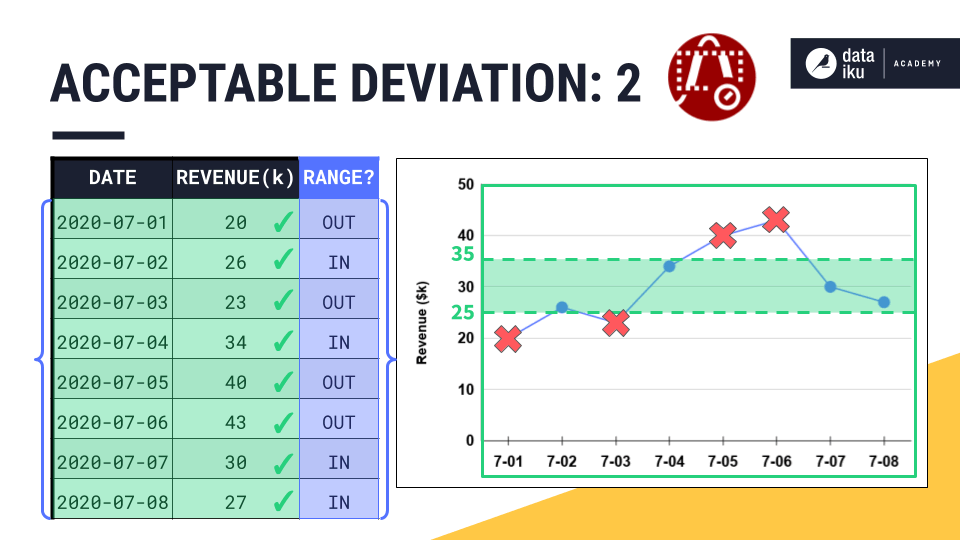### Minimal Segment Duration¶

Finally, let’s see how to define a minimal segment duration for retained time intervals.

While the acceptable deviation parameter gives us the flexibility to expand a valid time interval, the minimal segment duration parameter does just the opposite by imposing a minimum requirement on the duration of a valid interval.

In the short interval shown in the center below, all values lie within the threshold range. But perhaps, we require all intervals to be at least 7 days long. To enforce this requirement, we could set a minimal segment duration of 7 days, and thereby prevent intervals shorter than 7 days from being assigned an interval ID.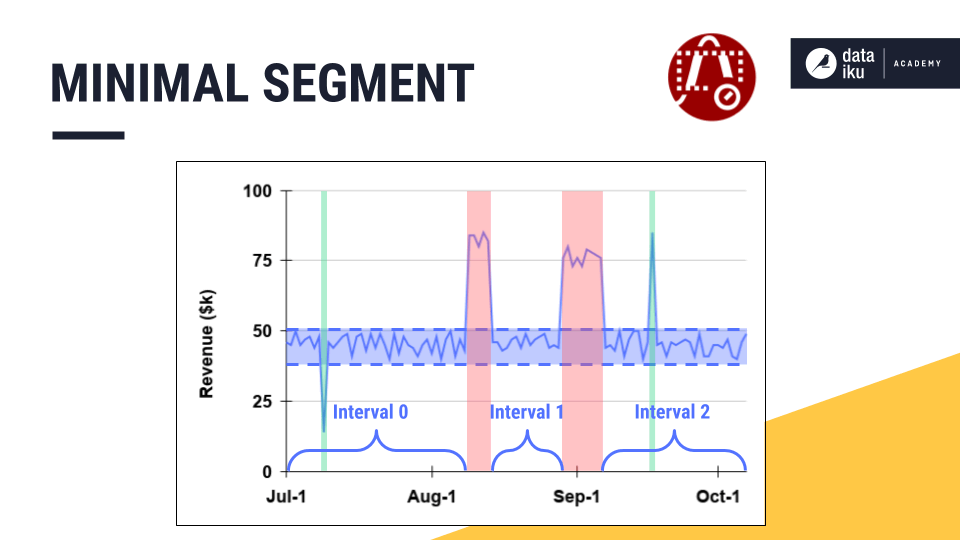Let’s see this in the table where the acceptable deviation and the minimal segment duration are both set to 0 days. The first two intervals (July 2nd and July 4th) include only one valid timestamp.

That makes their segment duration, or the difference between the first and the last valid timestamp, equal to 0 days.

If the minimal segment duration is 0, these single timestamps will remain separate interval IDs.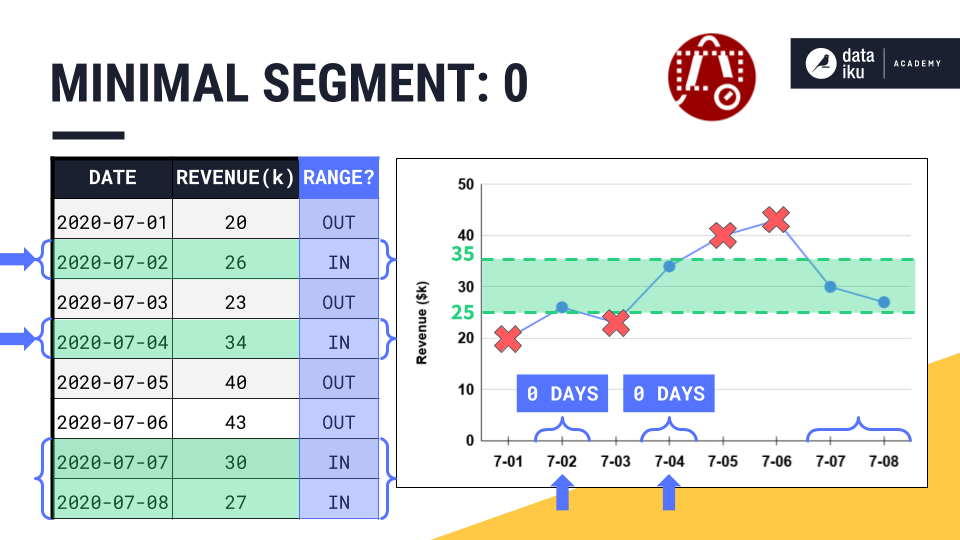But if we increase the minimal segment duration to 1 day, now these intervals are too short. They fail this requirement, and so Dataiku DSS will not assign them to an interval.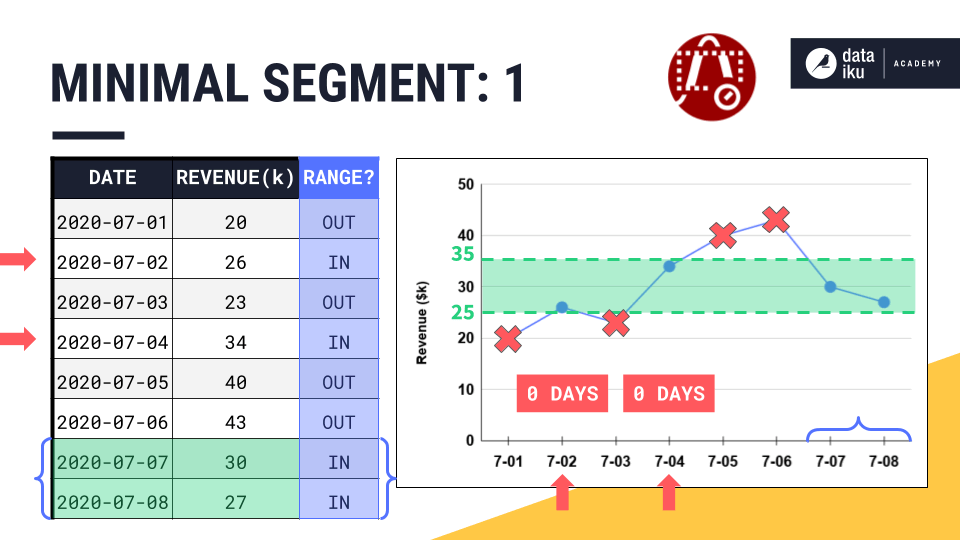## What’s next?¶

Now that you have a sense of the recipe’s intuition, dive into the recipe’s mechanics in Part 2!